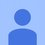# Solving linear equations under constraints

Given a matrix equation, $$AX=Y$$

$\begin{bmatrix} a_{11}&a_{12}&\dots&a_{1n} \\ a_{21}&a_{22}&\dots&a_{2n} \\ \vdots&\vdots&\ddots&\vdots\\ a_{m1}&a_{m2}&\dots&a_{mn} \\ \end{bmatrix}\times \begin{bmatrix} x_1\\ x_2\\ \vdots\\ x_n\\ \end{bmatrix}= \begin{bmatrix} y_1\\ y_2\\ \vdots\\ y_m\\ \end{bmatrix}$

with known $A$ and $Y$, and $m\ne n$, give a general method to solve for $X$, such that $0. Given that for the given equation(s) there is at least one solution under the given constraints. For multiple solutions any one will work (or maybe the one with least square after dealing with the constraints).

Additional constraints: Also consider the case of $m=n$, and that $A$ can be singular. Also assume, if necessary, $m and/or $m=1$ (ie. a single linear equation with multiple variables).

My approach: I already know about pseudoinverse and how to use them, but the problem with them is that they returns values outside the range. Consider with $A=\begin{bmatrix} 1&2&3 \end{bmatrix}$ and $X=\begin{bmatrix} 0.8\\ 0.9\\ 0.8 \end{bmatrix}$

What would be a good approach?Note by Sherlock Doyle
3 years, 3 months ago

This discussion board is a place to discuss our Daily Challenges and the math and science related to those challenges. Explanations are more than just a solution — they should explain the steps and thinking strategies that you used to obtain the solution. Comments should further the discussion of math and science.

When posting on Brilliant:

• Use the emojis to react to an explanation, whether you're congratulating a job well done , or just really confused .
• Ask specific questions about the challenge or the steps in somebody's explanation. Well-posed questions can add a lot to the discussion, but posting "I don't understand!" doesn't help anyone.
• Try to contribute something new to the discussion, whether it is an extension, generalization or other idea related to the challenge.
• Stay on topic — we're all here to learn more about math and science, not to hear about your favorite get-rich-quick scheme or current world events.

MarkdownAppears as
*italics* or _italics_ italics
**bold** or __bold__ bold
- bulleted- list
• bulleted
• list
1. numbered2. list
1. numbered
2. list
Note: you must add a full line of space before and after lists for them to show up correctly
paragraph 1paragraph 2

paragraph 1

paragraph 2

[example link](https://brilliant.org)example link
> This is a quote
This is a quote
    # I indented these lines
# 4 spaces, and now they show
# up as a code block.

print "hello world"
# I indented these lines
# 4 spaces, and now they show
# up as a code block.

print "hello world"
MathAppears as
Remember to wrap math in $$ ... $$ or $ ... $ to ensure proper formatting.
2 \times 3 $2 \times 3$
2^{34} $2^{34}$
a_{i-1} $a_{i-1}$
\frac{2}{3} $\frac{2}{3}$
\sqrt{2} $\sqrt{2}$
\sum_{i=1}^3 $\sum_{i=1}^3$
\sin \theta $\sin \theta$
\boxed{123} $\boxed{123}$

## Comments

Sort by:

Top Newest

It's more difficult than tutoriage. Maybe I could do this few years ago, but now forget about it. So, I also would like to know the answer.

- 3 years, 1 month ago

Log in to reply

×

Problem Loading...

Note Loading...

Set Loading...